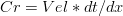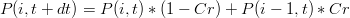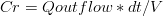# Modeling waterproperties transport

The steps that should be followed for modeling water properties transport are enumerated bellow.

Check the bathymetry:

```    1. See if there are channels "closed" by intertidal or land cells
```
```    2. Filter the bathymetry at least for the intertidal area
a. Create the Filter.dat input file
b. Run the FilterBathymetry.exe program
```

Use Module FillMatrix specifications to initialize all water properties. It is critical to know all the initialization options available.

Verify the options to compute transport processes:

```    1. Advection numerical scheme
```
```    2. Diffusion values
```
```    3. Open Boundary conditions
```
```    4. Atmospheric boundary condition
```
```    5. Land boundary condition
```
```    6. For particulate properties:
a. Settling velocity
b. Bottom boundary condition
```
```    7. Delete all the keywords that you aren't using.
Too many keywords make it difficult to read the input files and
to find errors.
```
```    1. In a 2D case choose a semi-implicit approach to compute the horizontal transport.
In the 3D case, an explicit one must be chosen due to stability problems;
```
```    2. By default the vertical transport is always implicit (don't change);
```
```    3. Identify in the horizontal grid the minimal horizontal spatial step (dx);
```
```    4. Identify an order of magnitude for the maximum velocity (Vel);
```
```    5. When the horizontal transport is computed explicitly, a first good approach is to
consider the Courant number(Cr) equal to 1:$Cr=Vel*dt/dx$

```
```    6. For the 2D case, with a semi-implicit approach, a higher Courant number can be tested;
```
```    7. In reality the Courant is a concept that works well in schematic tests in channels with
constant depth and velocity. In this case, an explicit upwind discretization of the
advection term of a property P becomes, for a velocity greather than zero:$P(i,t+dt)= P(i,t)*(1-Cr) + P(i-1,t)*Cr$
```
```    8. For real model applications, the Courant number based in the velocity is simplistic and
misleading. In reality the Courant number, in a conservative upwind discretization
of the advection term, is computed based in flows (Q) and volumes (V) in the 1D case is:$Cr = Q outflow * dt / V$
```

Common errors

```    1. Type of errors: Pontual instabilities in the mass balance
can occour usually in the wetting and drying process. These instabilities can have
several degrees. These degrees are enumerated in a descending order:
a) The most serious : the model crash in the transport of a conservative property
like salinity;
b) The model crash in computing the temperatura transport when the heat fluxes with
the atmosphere are computed;
c) The model crash when simulates properties with a settling velocity;
d) The model is able to compute the evolution of temperature (with heat fluxes)
and salinity but still persist some small instabilities that originate
i) wrong values of oxygen saturation and consequently wrong oxygen fluxes with the
atmosphere and the oxygen transport crash;
ii) Wrong biochemical rates that depend in the temperature and the transport
biochemical properties crash.

2. Possible solutions:
a) Filter the bathymetry. A steep bathymetry means drastic variations between
adjacent controle volumes and this way are created the conditions to occour
high courant numbers. This is dramatic in intertidal areas because very easily
in the wetting and drying process adjacent controle volume can have volume relations
of several orders of magnitude;
b) Decrease the time step;
c) Use the magic but very dangerous keywords 'MIN_VALUE' and 'MAX_VALUE' for each
property. In extreme cases you need to guarantee that all properties > 0
(MIN_VALE : 0.). The upper limitation (MAX_VALUE) is only needed in the salinity
and temperature. These two properties are critical because there are a lot
of rates that depend on this two properties. These magic keywords are dangerous
because when the model correct the field properties so there is any value < MIN_VALUE
and > MAX_VALUE the model generate mass in the first case and destroys in the second.
After each run is necessary to verify in the WaterProperties_x.fin5 hdf5 file the
mass_created and the mass_destroyed matrixes and verify the total mass created and
destroyed in the run. Be carefull in your analysis the values are
in Property x Volume.
```